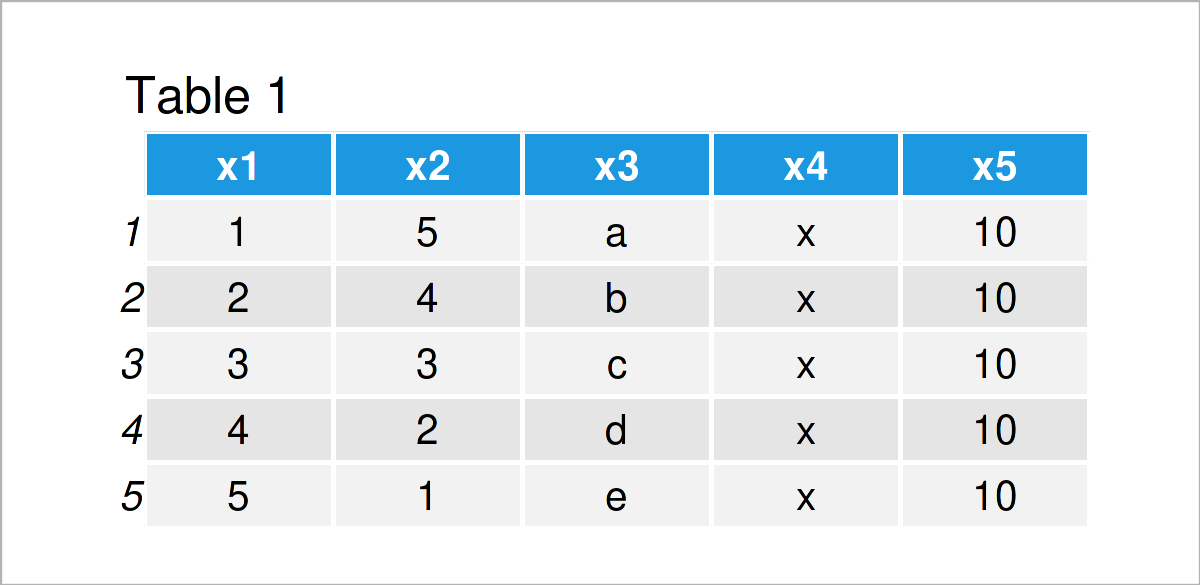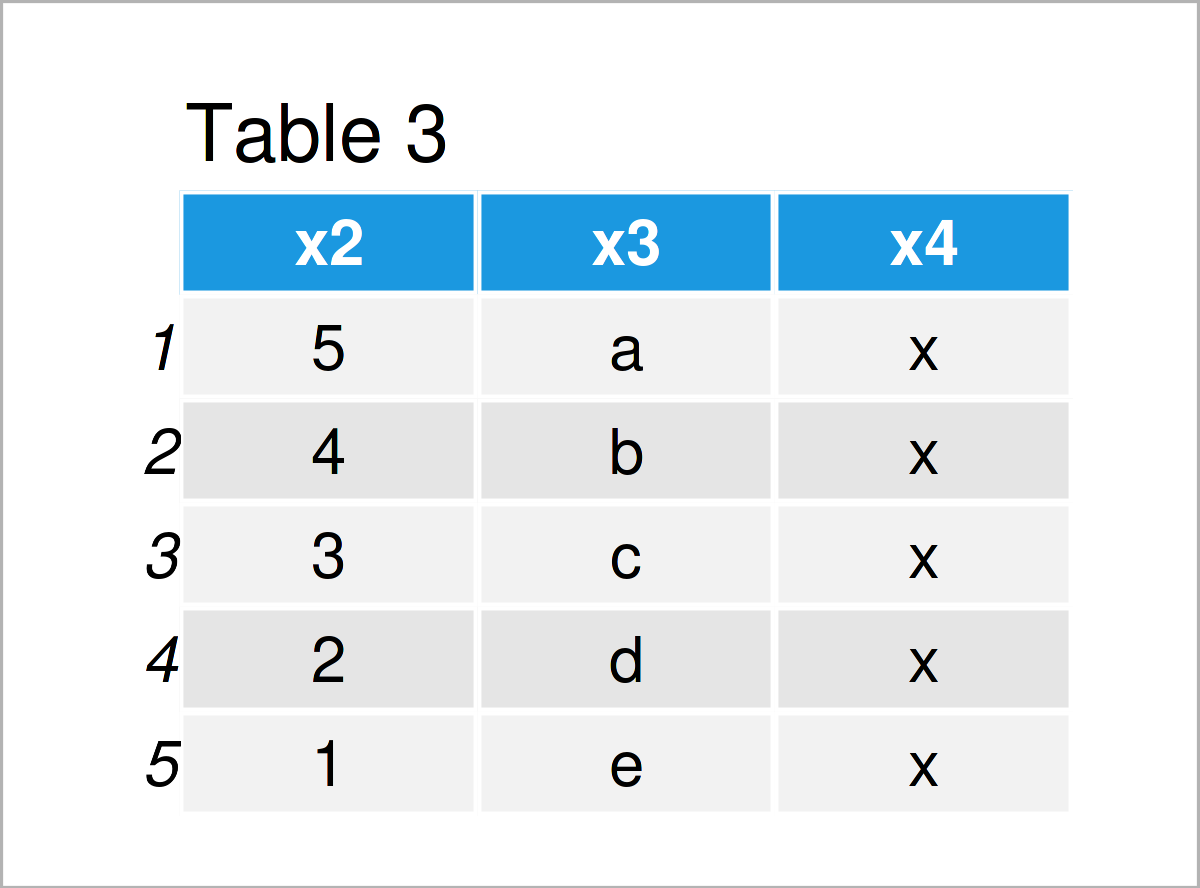# Create Data Frame from Another Existing Data Set in R (2 Examples)

In this article you’ll learn how to create a new data frame from existing data in the R programming language.

The content of the article is structured as follows:

## Creation of Example Data

The following data will be used as a basis for this R tutorial:

```data <- data.frame(x1 = 1:5, # Create example data frame x2 = 5:1, x3 = letters[1:5], x4 = "x", x5 = 10) data # Print example data frame```As you can see based on Table 1, our example data is a data frame object consisting of five rows and five variables.

## Example 1: Create Data Frame from Existing Data Using Column Names

Example 1 shows how to build up a new data frame based on the column names of another data frame.

To accomplish this, we can use square brackets and the c() function as shown below:

```data_new1 <- data[ , c("x1", "x2", "x5")] # Extract certain columns data_new1 # Print new data frame```Table 2 shows the output of the previous R syntax: A subset of our input data frame that was initialized based on the column names of the input data frame.

## Example 2: Create Data Frame from Existing Data Using Column Indices

In this example, I’ll illustrate how to make a data frame from another data set using the index values of the columns.

Similar to Example 1, we can use square brackets and the c() function for this task. However, this time we have to specify index numbers instead of variable names within the c() function:

```data_new2 <- data[ , c(2, 3, 4)] # Extract certain columns data_new2 # Print new data frame```Table 3 visualizes the output of the previous syntax: Another data frame subset.

## Video & Further Resources

If you need further explanations on the R programming codes of this tutorial, I recommend watching the following video on my YouTube channel. In the video, I show the R programming codes of this article:

Additionally, you may have a look at some of the related tutorials on my website. Some posts that are related to the creation of a new data frame from existing data can be found below: